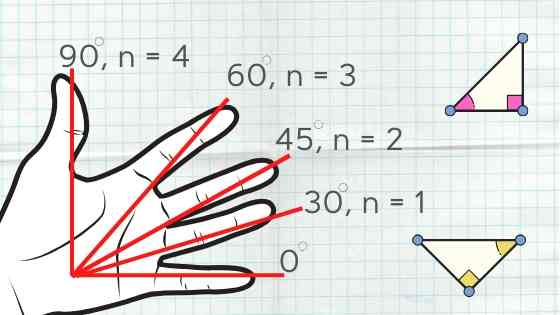# Trigonometry On FingersTrigonometry is one of the most widely used sec...

As you may already know, trigonometry is a branch of mathematics that studies the relationship between side lengths and angles of triangles.There are three main functions used in trigonometry: sine, cosine, tangent, which are usually labelled as sin, cos, tan respectively.
Let’s review what each one of the above functions does. Let’s also learn how to do trigonometry on fingers.

In the non-right angle triangles we use Sine Law and Cosine Law to determine the side lengths and angles.

Trigonometry is widely used in many aspects of our life, especially in construction, astronomy, physics, electronic music, flight engineering, marine engineering, navigation, medicine, cartography, electricity, etc.
Let’s take a look at one of the practical usages of trigonometry in our life.

#### Problem:

Your house has a roof which makes up a triangle as shown below.

You need to calculate the lengths of the roof slopes AB and BC when the angles of elevation A and C are given. The measure of angle B = 180o – (A + B) = 180o – (35o + 41o) = 104o.

Now let’s have a look at another trigonometry problem we usually come across at school.
We often need to calculate the values of trigonometric functions, like sin 41, cos 30, tan 90, sin 45, …. sin X, cos X, tan X and so on.
It is not that trivial to evaluate the values for these functions for any angle, but there are some of them, which are very common and are frequently used. The most common angles are: 0, 30, 45, 60 and 90 and for these angles we usually have to memorize the table of “Trigonometric Ratios of Standard Angles” which could be overwhelming and seem like a ‘rocket science’.

The good news is, that you can use your left hand fingers to find the ratio for sine and cosine functions easily.

Below is a good illustration of what we have just discovered.

Try it for yourself and check your result in the table of “Trigonometric Ratios of Standard Angles” given above.
It is almost the same, but the positioning should start from the thumb.

Try finding the cosine ratios for the standard angles using your left hand fingers and check the results in the above table.